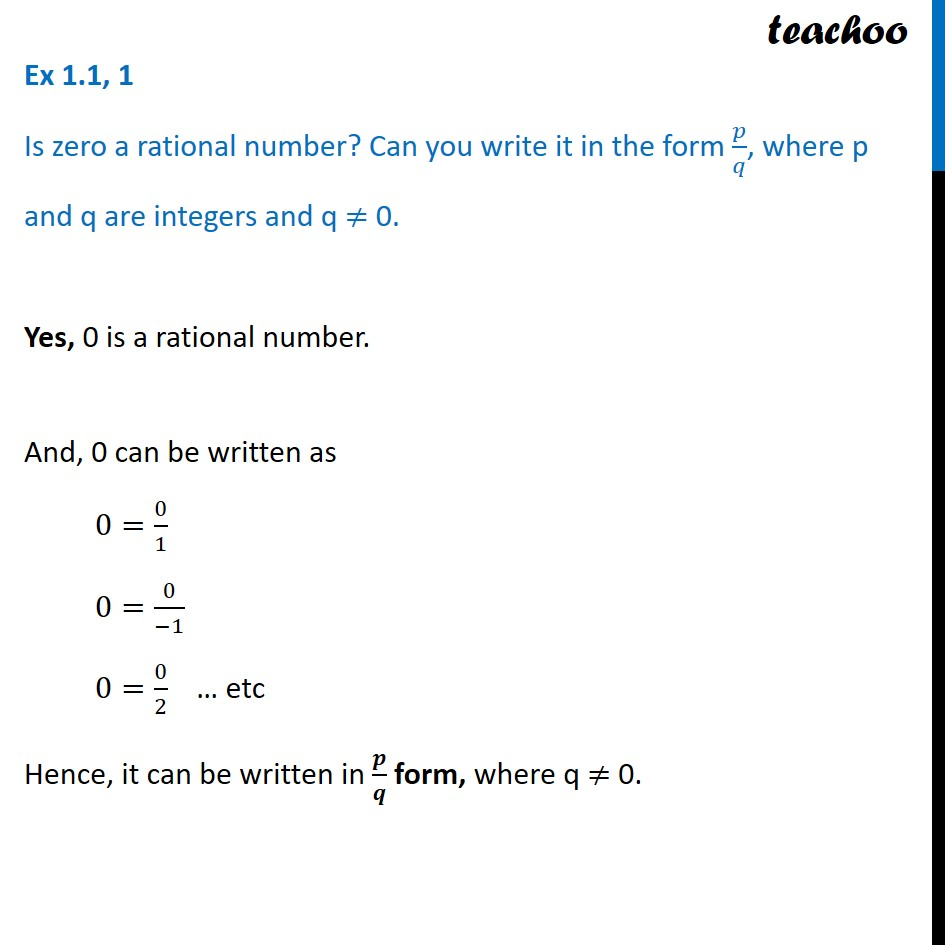Ex 1.1

Chapter 1 Class 9 Number Systems
Serial order wiseGet live Maths 1-on-1 Classs - Class 6 to 12

### Transcript

Ex 1.1, 1 Is zero a rational number? Can you write it in the form 𝑝/𝑞, where p and q are integers and q ≠ 0.Yes, 0 is a rational number. And, 0 can be written as 0=0/1 0=0/(−1) 0=0/2 … etc Hence, it can be written in 𝒑/𝒒 form, where q ≠ 0.